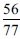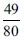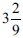# User ForumSubject :IMO    Class : Class 7

Simplify :ABCDNone of these

## Ans 1:

Class : Class 8
[{(11/4) / (11/6)} / (7/8)] * (7/12) + (5/7) / (9/28) = {(6/4) / (7/8)} * (7/12) + (20/9) = (12/7) * (7/12) + (20/9) = 1 * (20/9) = 20/9 = 3 2/9 Thus, answer is C